A Comparison of a Pair of Sixes

It is often assumed that the better product costs more but is that always true?  Well, hopefully, this comparison may answer that question, at least as it pertains to loudspeakers.

The two speakers used are both 6 inch units.  One currently sells for \$22 and the other currently sells for just under \$200.  They are designated here as 22d and 200d. so there are no mug shots of the usual suspects.  There is a photo of the test box used.  It has an internal volume of 294 in^3, it is a sealed box and is stuffed with 60g of untreated, other than washed, Australian wool.

The 200d is a full range and the 22d is a mid range.  This test uses both as mid range, despite that the 200d does very well to about 15khz and would work exceptionally well in a two way system covering the band from 300hz and up in a sealed box of volume = 274 in^3 with a Qtc= 0.707  A second order 300hz high pass filter is recommended to keep low frequency energy from entering the voice coil and increasing diaphragm excursion thus increasing modulation distortion without producing any appreciable low frequency output.

The 22d will work well in a sealed box of just over 1 ft^3 (to be exact, 1760 in^3).  Again, a second order 300hz high pass filter is recommended  and for the same reasons stated above.

According to BBP (BassBoxPro), the both will perform well in a sealed boxes as above down to 150hz, again with the appropriate filter.  The 22d will need a tweeter tom carry the band above 5khz.

Here are some of the differences between the drivers.  The 30W condition on the 200d is rather interesting. It limits the frequency range to 30W above 200hz.  Based on that, it is difficult to accept the claim that the 20d can handle 40W all the way to 80hz.

TABLE 1

 22d 22d  comment 200d 200d comment VC diameter 1" 1.375" Xmax 2mm 6mm Power handling 40W 30W 2nd order hi pass filter 200hz Frequency range 80-20000 specified by plot and text specified by plot only

The sealed and open baffles

 PHOTO 1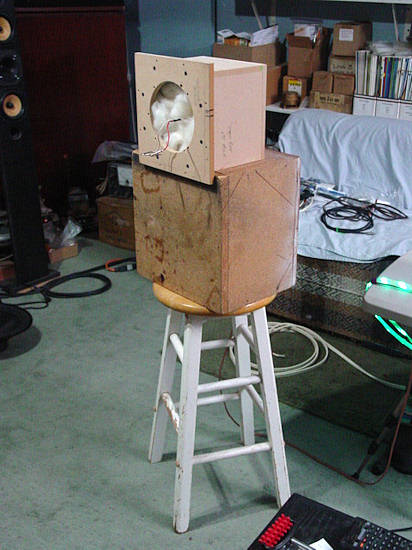PHOTO 2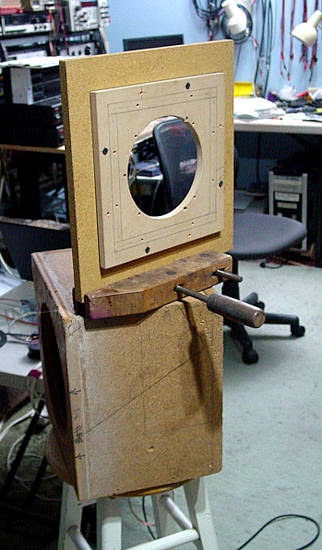The two figures, 1 & 2 below, show the frequency response measured at 1w1m in a sealed box of 294 in^3.  The lower grey trace is THD (Total Harmonic Distortion)  Minus 40dB (-40dB) is about 1% THD and -45dB is 0.6%  It seems the 22d produces less THD and it should be added that this doesn't imply that the better sounding unit will be the one with less THD, SEE  http://www.sengpielaudio.com/calculator-thd.htm

 FIGURE 1  22d FIGURE 2  200d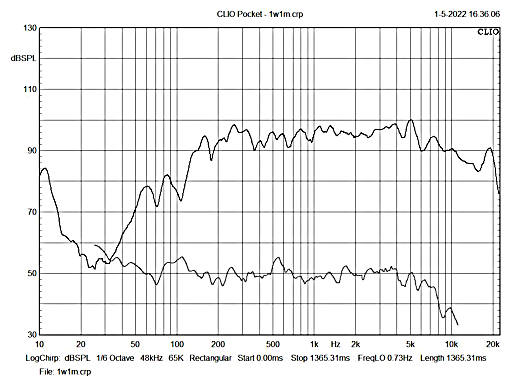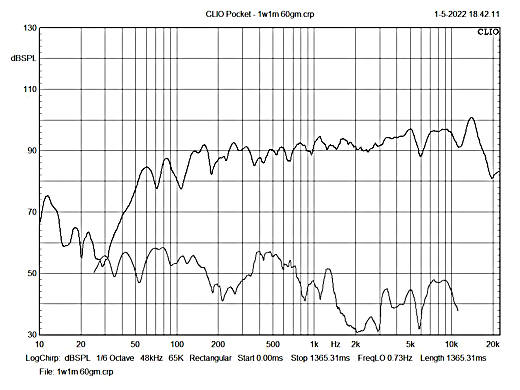For those of you who prefer an open (flat) baffle, here's a set of response curves taken with the speakers mounted on a 12" x 13" flat baffle.  Both begin to fall at 500hz.  If -3dB is taken as the knee, that point is around 450hz   Under pure anechoic conditions, this baffle would have its knee around 1khz but rooms are reverberant fields so that theorem goes to hell in a handcart, PDQ (Pretty Damn Quick)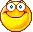Under these conditions, the two curves bear a stark resemblance.  The 22d shows more low frequency distortion and may be due to its plain paper cone; the 200d has an exotic material impregnated cone and is more stable in that it has less tendency to deform.  However, the high distortion peak between 900hz and 1500hz isn't understood.  A S.W.A.G. (Scientific Wild Ass Guess) would be that the 200d doesn't have a whizzer cone whereas the 22d does.  Both do, however, have curvilinear cones. FIGURE 3  22d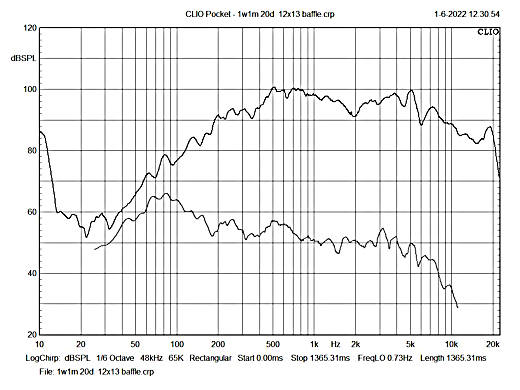FIGURE 4  200d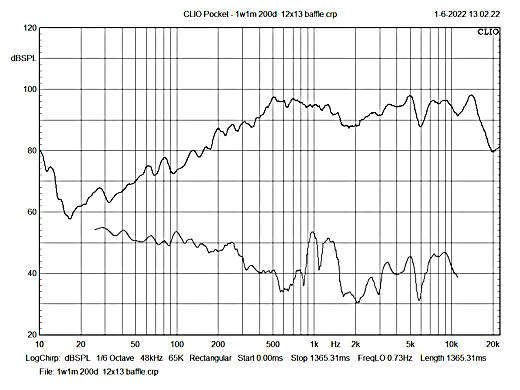IMPEDANCE:  BLACK=22d;  RED=200d The impedance of the 22d speaker at 10,200hz is 14W and that of the 200d is about 7W (ref figs 19-21)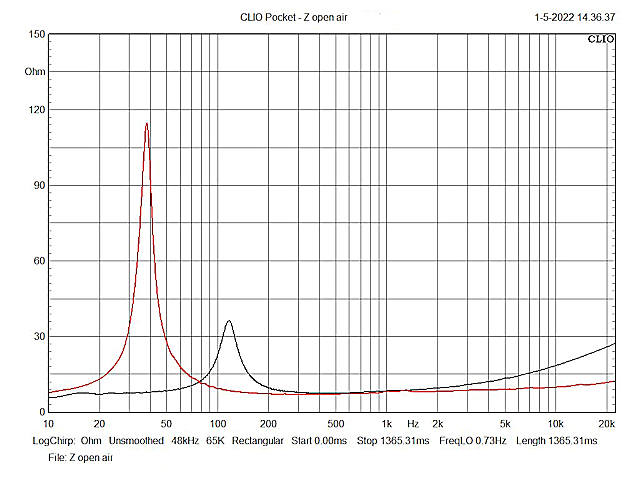This addition was an afterthought, hence no figure designation These next spectrographs were all done with the speakers in a sealed box at 2.83 volts and at the frequency specified.  This corresponds to a power transfer at 8W.  However, the 22d speaker impedance at that frequency is 14W, which is a power transfer of 0.572W.  One watt would here require 3.74V.  The difference is 2.42dB.  Now, additional power would imply a higher distortion.  As it works out, the distortion at 10,200hz at a half watt is 0.316% and at one watt it's 0.413%, a rise of about one tenth of 1%. The frequencies were initially set to start at the speakers' mid range lower frequency, that being 300hz.  It was initially considered to move up the spectrum in octaves but it was thought to produce redundancy.  For example, the second harmonic at 300hz is 600hz, the third is 1200hz, etc, but the second harmonic of 600hz is also 1200hz.  After some thought, a Fibonacci series was selected, beginning at 300hz.  The second term also being 300hz was omitted for obvious reasons.  The rest of the series should also be obvious.  This looked good as no term in the series is a whole number multiple of any other, except the second term.  It just looked good.  So, there.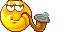The following figures show the fundamental and associated harmonics for each speaker and are placed side by side for easy comparison.

 Seems the 22d has less harmonic distortion at 300hz than the more expensive 200d.  Referring to figs 1 & 2, we can see that the second harmonic of the 22d is 46dB below the fundamental.  The second harmonic of the 200d is 43dB below its fundamental.  these equate to 0.501% and 0.708%, resp.  Now, we come to the thirdn harmonic at 900hz.  Here we have a difference of 14dB (54dB-40dB), showing that at 900hz, the 200d has 0.8% more distortion at 900hz. This process can be repeated for every harmonic and can be very tedious if done manually.  A distortion analyzer is highly recommended.  Thanks to the microprocessor, the CLIO has such included, thus computing this at a mind boggling speed.  The CLIO also will show 2nd and 3rd harmonic distortion levels as well as THD.  That little box wasn't cheap. Another device here is the PICO Scope, which also has a spectrum analyzer.  This one shows the voltage at the peak, thus making it easier to manually compute distortion.  The CLIO was preferred here as it has correction algorithms for the supplied microphone.  With the PICO, the mic used there would have to be calibrated along with the mic pre-amp used.  Microphone calibrators can be very expensive.  Typically, though, any decent lab mic will have far less distortion than any loudspeaker and the CLIO can be used to measure the distortion of the mic pre-amp. For more on calculating THD, see http://www.sengpielaudio.com/calculator-thd.htm FIGURE 5  22d  300hz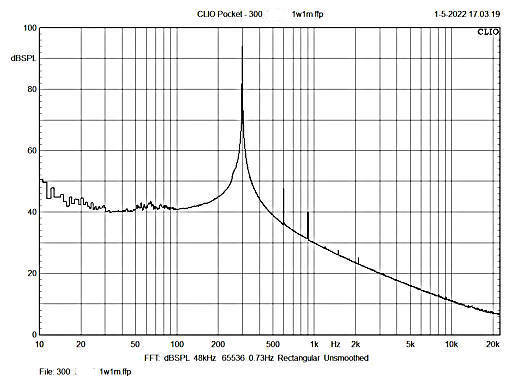FIGURE 6  200d  300hz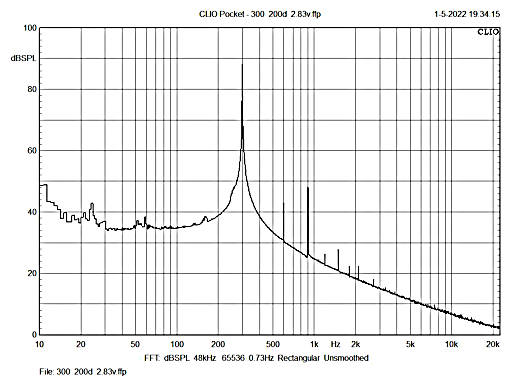FIGURE 7  22d  600hzFIGURE 8  200d  600hz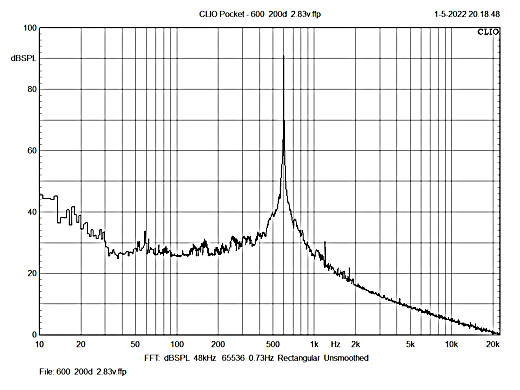FIGURE 9  22d  900hz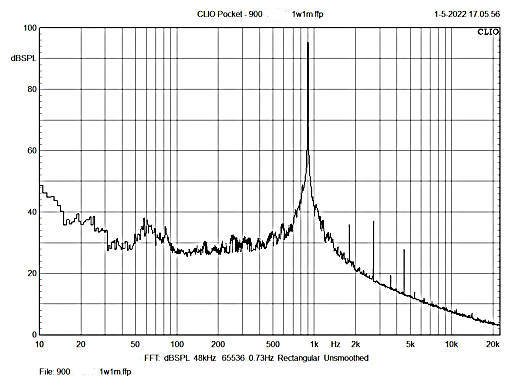FIGURE 10  200d  900hz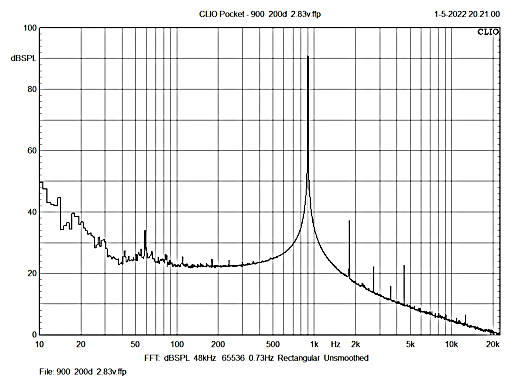FIGURE 11  22d  1500hz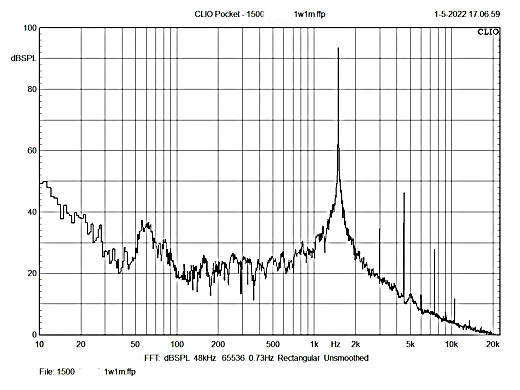FIGURE 12  200d  1500hz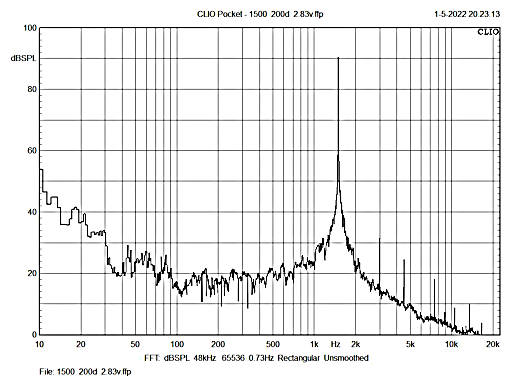FIGURE 13  22d  2400hz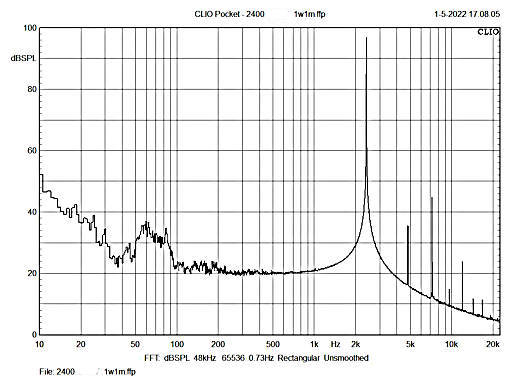FIGURE 14  22d  2400hz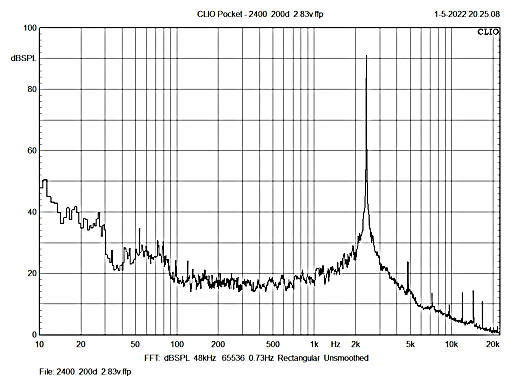FIGURE 15  22d  3900hz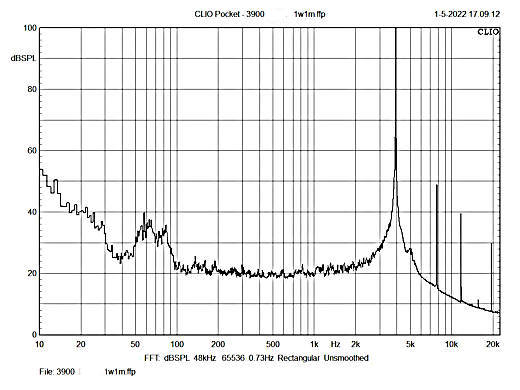FIGURE 16  200d  3900hz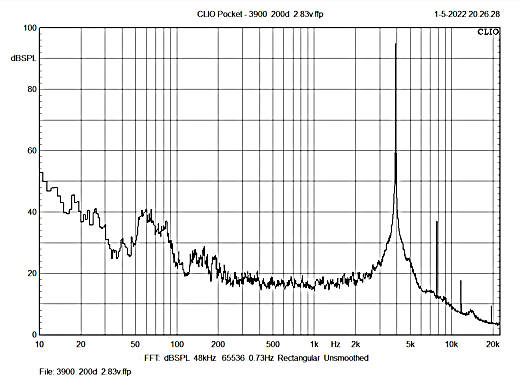FIGURE 17  22d  6300hz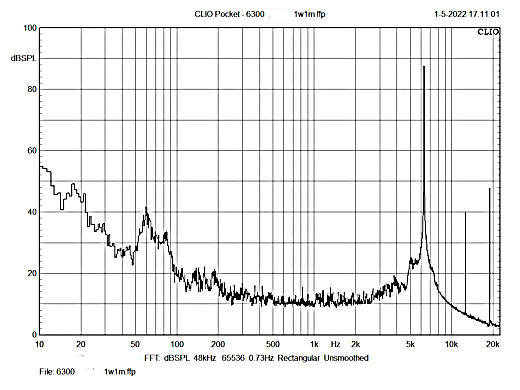FIGURE 18  200d  6300hz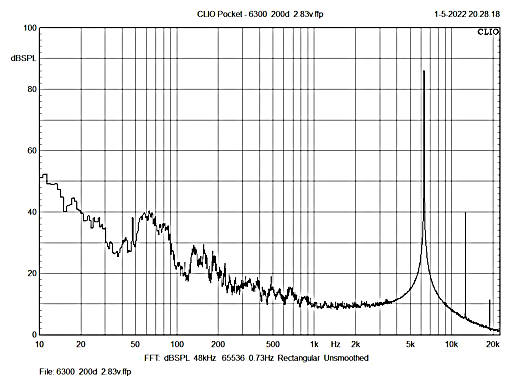FIGURE 19  22d  10200hz In this figure, notice the SPL at 10,200hz.  It's about 87dB and the harmonic is at 37dB, a difference of 50dB and a distortion content of 0.316%. With 2.83v applied, the power transfer will be 0.572 watt.  Compare this to the same fundamental frequency and its harmonic in figure 3, which is compiled from two sweeps of 1 second each.  The generator is a constant voltage output, so as the impedance rises, the current drops as does the power transfer and the SPL.Figure 21 elaborates on this in a little more detail.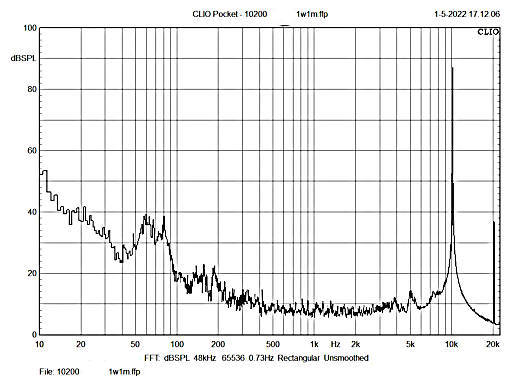FIGURE 20  200d  10200hz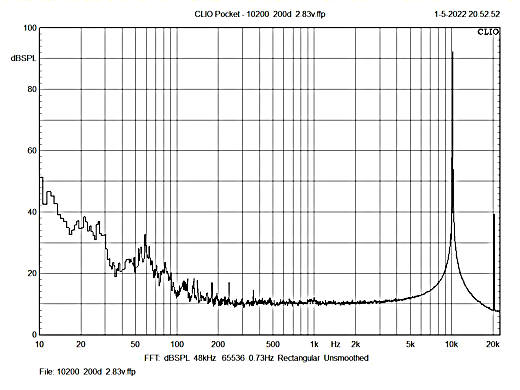FIGURE 21  22d  10200hz The fundamental here is with 3.74V applied, (1 watt).  The fundamental rises to 89.5dB and the harmonic rises to 47.5dB, with a distortion content of 0.418%, a distortion increase of about 1/10th of 1%.  Taking this to 10 watts was considered but but at 100dB, 10khz is somewhat irritating to the ear due to its takeing several seconds for the analyzer to reach all its peaks to get an accurate measurement.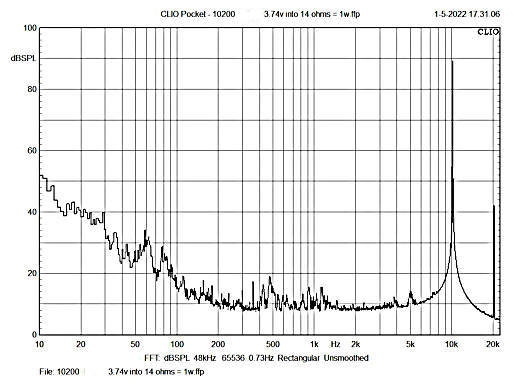Conclusion The above data may show that which appears to be very subtle differences, however, that data doesn't have ears. The only way to determine a difference is to listen to the two speakers under identical conditions.  The best that could be achieved here was to record the sound and place the file here.  So, let it be recorded; so let it be played. (A variation of a line in Cecil B. DeMille's epic 1956 movie The Ten Commandments when, after agreeing to a policy, the Pharaoh (Yul Brynner) would say, "So let it be written, so let it be done.")Anyway, here are two soundtracks played with a 2 second break between them.  The first is that of the 22d and the second is that of the 200d.  Both are recorded with a digital recorder which was placed about 18" from the speaker.  The speakers were mounted in turn into the same 300 in^3 box stuffed with 60g wool.  The recording was second order band pass filtered at 300hz and 5000hz to cover only the midrange.Here are two response curves. The left one is a sweep and the right one is an FFT analysis of pink noise, both filtered as described above.  In both, the red trace is that of the 22d and the green trace is that of the 200d.The link to the SOUNDTRACK is at the bottom.              FIGURE 22                                                                                                                   FIGURE 23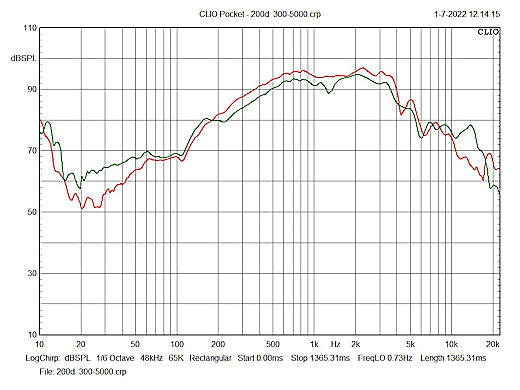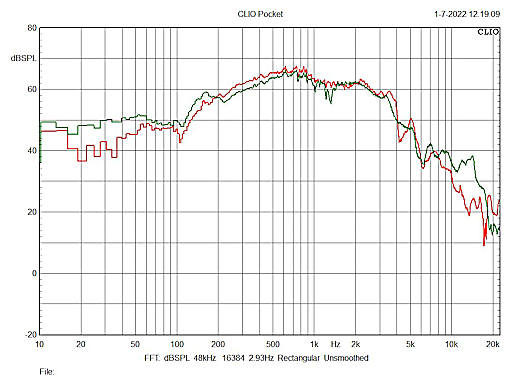SOUNDTRACK

Back to the loudspeaker main page